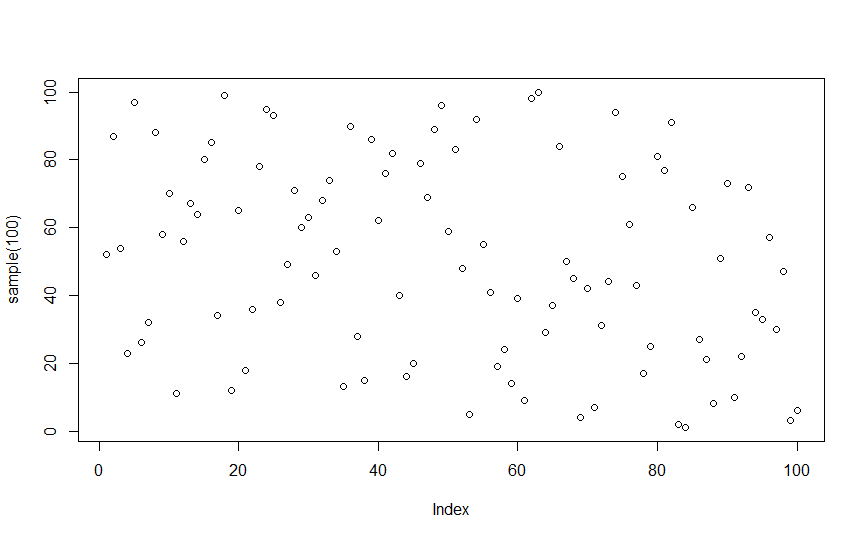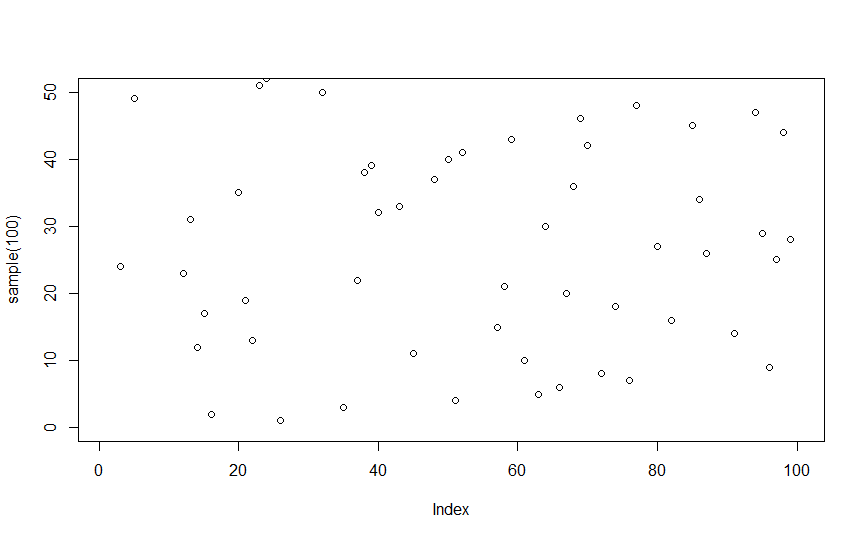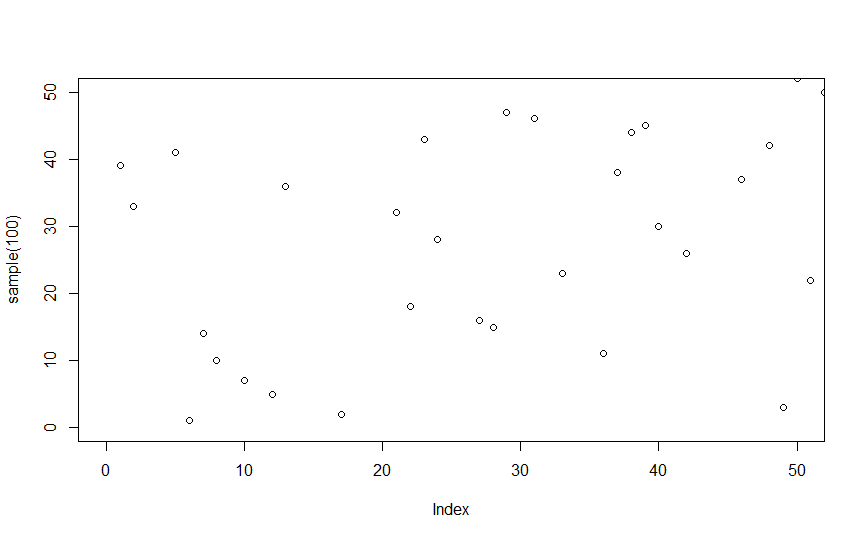# ylim() and xlim() in R

Graphs can provide excellent insight into data and help understand the relationships in the dataset. R is such a powerful tool for data analysis has many functions like `ggplot()`, `plot()`, and many more, which can help make beautiful, useful graphs and many types.

Sometimes, one might need to make sure that the plot lies between certain values and not exceed these values. Two functions that can be used in such situations are the `ylim()` and `xlim()` functions. Both these functions are used to set the lower and upper limit on the y-axis and x-axis, respectively.

Let’s start with the `ylim()` function. It specifies the upper and lower limit of the y-axis. It is a fundamental function and can be used inside the `ggplot()`, `plot()`, and other plot functions as a parameter. In the example below, we will first plot a basic scatter plot of random distribution and then set the lower limit of the y-axis as 0 and the upper limit as 50 using `ylim()`.

``````plot(sample(100))
````````````plot(sample(100), ylim = c(0,50))
``````Notice the change in the y-axis after setting the upper and lower limit.

Similarly we can use the `xlim()` function for x-axis. For example:

``````plot(sample(100), ylim = c(0,50), xlim = c(0,50))
``````Contribute
DelftStack is a collective effort contributed by software geeks like you. If you like the article and would like to contribute to DelftStack by writing paid articles, you can check the write for us page.

## Related Article - R Plot

• The scale_x_discrete Function in R
• Set Thickness of Plots in R